Documentation

### This is machine translation

Mouseover text to see original. Click the button below to return to the English version of the page.

Note: This page has been translated by MathWorks. Click here to see
To view all translated materials including this page, select Country from the country navigator on the bottom of this page.

## Vibration Control in Flexible Beam

This example shows how to tune a controller for reducing vibrations in a flexible beam.

### Model of Flexible Beam

Figure 1 depicts an active vibration control system for a flexible beam.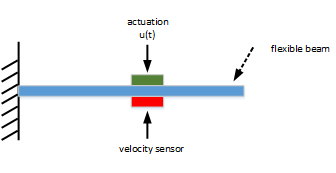Figure 1: Active control of flexible beam

In this setup, the actuator delivering the forceand the velocity sensor are collocated. We can model the transfer function from control inputto the velocityusing finite-element analysis. Keeping only the first six modes, we obtain a plant model of the form

``

with the following parameter values.

```% Parameters xi = 0.05; alpha = [0.09877, -0.309, -0.891, 0.5878, 0.7071, -0.8091]; w = [1, 4, 9, 16, 25, 36]; ```

The resulting beam model foris given by

```% Beam model G = tf(alpha(1)^2*[1,0],[1, 2*xi*w(1), w(1)^2]) + ... tf(alpha(2)^2*[1,0],[1, 2*xi*w(2), w(2)^2]) + ... tf(alpha(3)^2*[1,0],[1, 2*xi*w(3), w(3)^2]) + ... tf(alpha(4)^2*[1,0],[1, 2*xi*w(4), w(4)^2]) + ... tf(alpha(5)^2*[1,0],[1, 2*xi*w(5), w(5)^2]) + ... tf(alpha(6)^2*[1,0],[1, 2*xi*w(6), w(6)^2]); G.InputName = 'uG'; G.OutputName = 'y'; ```

With this sensor/actuator configuration, the beam is a passive system:

```isPassive(G) ```
```ans = logical 1 ```

This is confirmed by observing that the Nyquist plot ofis positive real.

```nyquist(G) ```### LQG Controller

LQG control is a natural formulation for active vibration control. The LQG control setup is depicted in Figure 2. The signalsandare the process and measurement noise, respectively.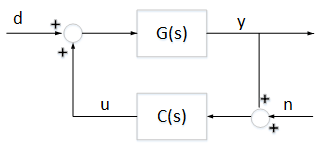Figure 2: LQG control structure

First use `lqg` to compute the optimal LQG controller for the objective

``

with noise variances:

``
```[a,b,c,d] = ssdata(G); M = [c d;zeros(1,12) 1]; % [y;u] = M * [x;u] QWV = blkdiag(b*b',1e-2); QXU = M'*diag([1 1e-3])*M; CLQG = lqg(ss(G),QXU,QWV); ```

The LQG-optimal controller `CLQG` is complex with 12 states and several notching zeros.

```size(CLQG) ```
```State-space model with 1 outputs, 1 inputs, and 12 states. ```
```bode(G,CLQG,{1e-2,1e3}), grid, legend('G','CLQG') ```Use the general-purpose tuner `systune` to try and simplify this controller. With `systune`, you are not limited to a full-order controller and can tune controllers of any order. Here for example, let's tune a 2nd-order state-space controller.

```C = ltiblock.ss('C',2,1,1); ```

Build a closed-loop model of the block diagram in Figure 2.

```C.InputName = 'yn'; C.OutputName = 'u'; S1 = sumblk('yn = y + n'); S2 = sumblk('uG = u + d'); CL0 = connect(G,C,S1,S2,{'d','n'},{'y','u'},{'yn','u'}); ```

Use the LQG criterionabove as sole tuning goal. The LQG tuning goal lets you directly specify the performance weights and noise covariances.

```R1 = TuningGoal.LQG({'d','n'},{'y','u'},diag([1,1e-2]),diag([1 1e-3])); ```

Now tune the controller `C` to minimize the LQG objective.

```[CL1,J1] = systune(CL0,R1); ```
```Final: Soft = 0.478, Hard = -Inf, Iterations = 40 ```

The optimizer found a 2nd-order controller with. Compare with the optimalvalue for `CLQG`:

```[~,Jopt] = evalGoal(R1,replaceBlock(CL0,'C',CLQG)) ```
```Jopt = 0.4673 ```

The performance degradation is less than 5%, and we reduced the controller complexity from 12 to 2 states. Further compare the impulse responses fromtofor the two controllers. The two responses are almost identical. You can therefore obtain near-optimal vibration attenuation with a simple second-order controller.

```T0 = feedback(G,CLQG,+1); T1 = getIOTransfer(CL1,'d','y'); impulse(T0,T1,5) title('Response to impulse disturbance d') legend('LQG optimal','2nd-order LQG') ```### Passive LQG Controller

We used an approximate model of the beam to design these two controllers. A priori, there is no guarantee that these controllers will perform well on the real beam. However, we know that the beam is a passive physical system and that the negative feedback interconnection of passive systems is always stable. So ifis passive, we can be confident that the closed-loop system will be stable.

The optimal LQG controller is not passive. In fact, its relative passive index is infinite becauseis not even minimum phase.

```getPassiveIndex(-CLQG) ```
```ans = Inf ```

This is confirmed by its Nyquist plot.

```nyquist(-CLQG) ```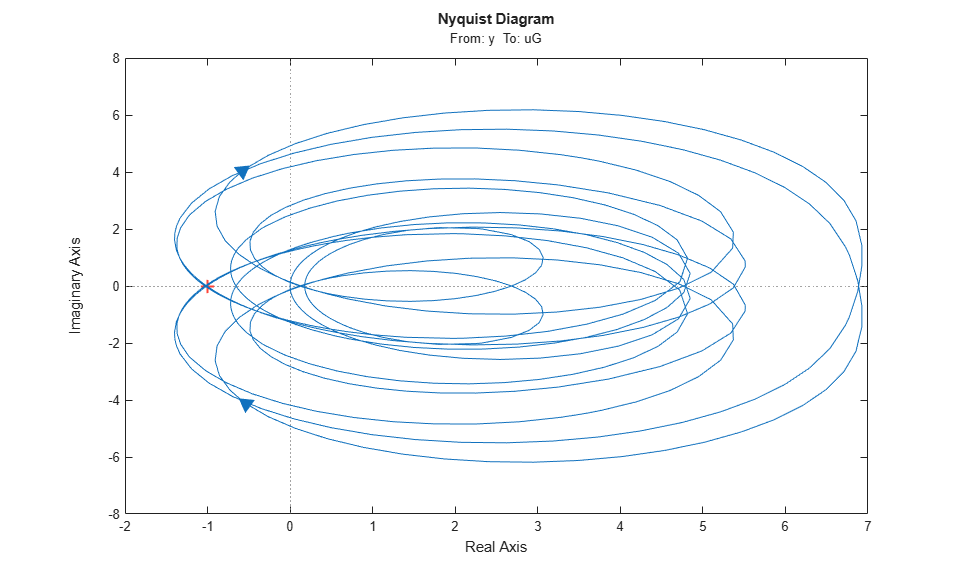Using `systune`, you can re-tune the second-order controller with the additional requirement thatshould be passive. To do this, create a passivity tuning goal for the open-loop transfer function from `yn` to `u` (which is). Use the "WeightedPassivity" goal to account for the minus sign.

```R2 = TuningGoal.WeightedPassivity({'yn'},{'u'},-1,1); R2.Openings = 'u'; ```

Now re-tune the closed-loop model `CL1` to minimize the LQG objectivesubject tobeing passive. Note that the passivity goal `R2` is now specified as a hard constraint.

```[CL2,J2,g] = systune(CL1,R1,R2); ```
```Final: Soft = 0.478, Hard = 1, Iterations = 37 ```

The tuner achieves the samevalue as previously, while enforcing passivity (hard constraint less than 1). Verify thatis passive.

```C2 = getBlockValue(CL2,'C'); passiveplot(-C2) ```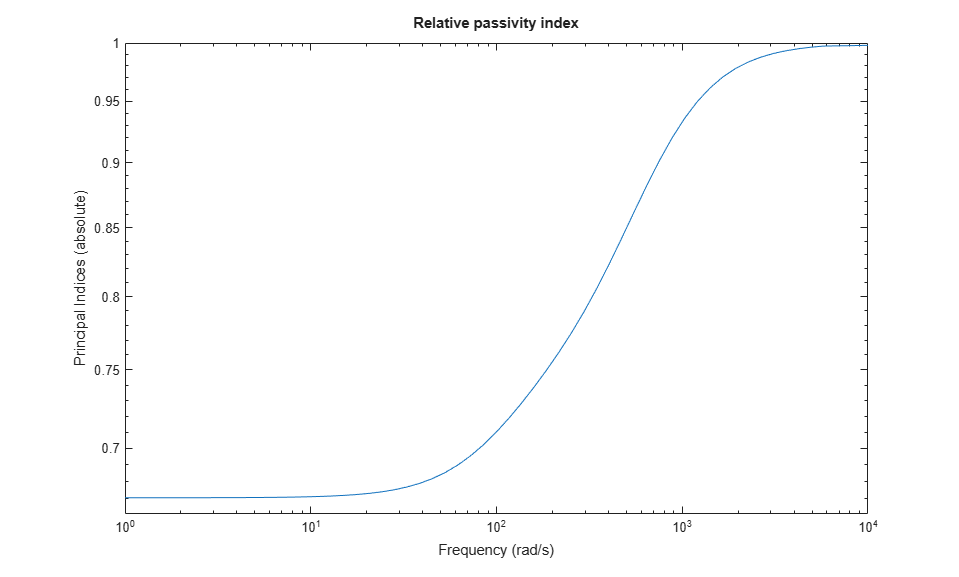The improvement over the LQG-optimal controller is most visible in the Nyquist plot.

```nyquist(-CLQG,-C2) legend('LQG optimal','2nd-order passive LQG') ```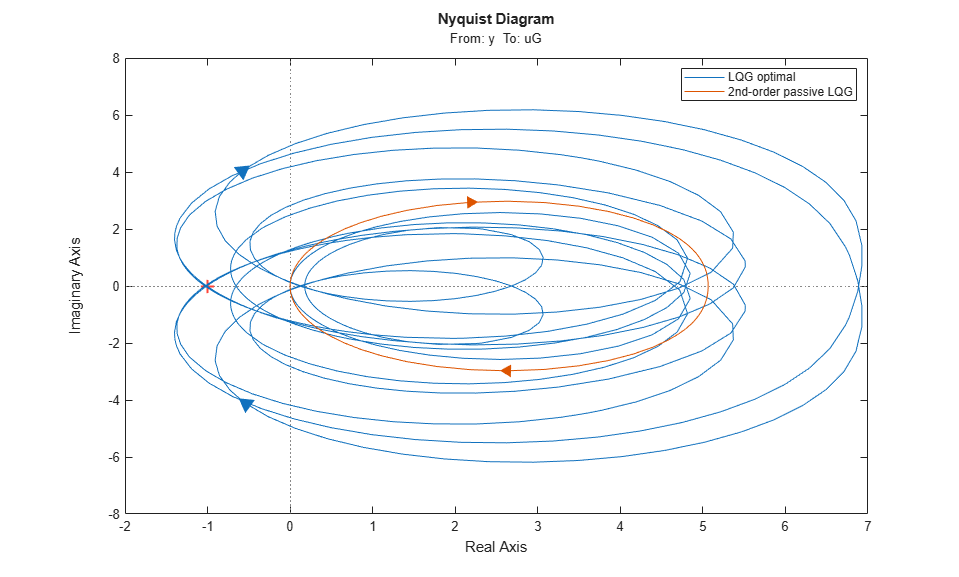Finally, compare the impulse responses fromto.

```T2 = getIOTransfer(CL2,'d','y'); impulse(T0,T2,5) title('Response to impulse disturbance d') legend('LQG optimal','2nd-order passive LQG') ```Using `systune`, you designed a second-order passive controller with near-optimal LQG performance.

## Related Topics

#### Learn how to automatically tune PID controller gains

Download code examples